Documentation

### This is machine translation

Mouseover text to see original. Click the button below to return to the English version of the page.

## Design Optimization of a Six-element Yagi-Uda Antenna

This example optimizes a 6-element Yagi-Uda antenna for both directivity and $300\Omega$ input match using a global optimization technique. The radiation patterns and input impedance of antennas are sensitive to the parameters that define their shapes. The multidimensional surface over which such optimizations must be performed have multiple local optima. This makes the task of finding the right set of parameters satisfying the optimization goals particularly challenging and requires the use of global optimization techniques. One such technique is pattern search, a direct search based optimization technique that has yielded impressive results for antenna design optimization.

The Yagi-Uda antenna is a widely used radiating structure for a variety of applications in commercial and military sectors. This antenna has been popular for reception of TV signals in the VHF-UHF range of frequencies . The Yagi is a directional traveling-wave antenna with a single driven element, usually a folded dipole or a standard dipole, which is surrounded by several passive dipoles. The passive elements form the reflector and director. These names identify the positions relative to the driven element. The reflector dipole is behind the driven element in the direction of the back lobe of the antenna radiation, while the director is in front of the driven element, in the direction where a main beam forms.

### Design Parameters

Choose initial design parameters in the center of the VHF band . The datasheet lists a $50\Omega$ input impedance after taking into account a balun. Our model does not account for the presence of the balun and therefore will match to the typical folded dipole input impedance of $300\Omega$.

```fc = 165e6; wirediameter = 12.7e-3; c = physconst('lightspeed'); lambda = c/fc; Z0 = 300; BW = 0.1*fc; fmin = fc - 2*(BW); fmax = fc + 2*(BW); Nf = 101; freq = linspace(fmin,fmax,Nf);```

### Create Yagi-Uda Antenna

The driven element for the Yagi-Uda antenna is a folded dipole. This is a standard exciter for such an antenna. Adjust the length and width parameters of the folded dipole. Since we model cylindrical structures as equivalent metal strips, the width is calculated using a utility function available in the Antenna Toolbox™. The length is chosen to be $\lambda /2$ at the design frequency.

```d = dipoleFolded; d.Length = lambda/2; d.Width = cylinder2strip(wirediameter/2); d.Spacing = d.Length/60;```

Create a Yagi-Uda antenna with the exciter as the folded dipole. Choose the reflector and director length to be $\lambda /2$. Set the number of directors to four. Choose the reflector and director spacing to be $0.3\lambda$, $0.25\lambda$ respectively. These choices are an initial guess and will serve as a start point for the optimization procedure. Show the initial design.

```Numdirs = 4; refLength = 0.5; dirLength = 0.5*ones(1,Numdirs); refSpacing = 0.3; dirSpacing = 0.25*ones(1,Numdirs); exLength = d.Length/lambda; exSpacing = d.Spacing/lambda; initialdesign = [refLength dirLength refSpacing dirSpacing exLength exSpacing].*lambda; yagidesign = yagiUda; yagidesign.Exciter = d; yagidesign.NumDirectors = Numdirs; yagidesign.ReflectorLength = refLength*lambda; yagidesign.DirectorLength = dirLength.*lambda; yagidesign.ReflectorSpacing = refSpacing*lambda; yagidesign.DirectorSpacing = dirSpacing*lambda; show(yagidesign)```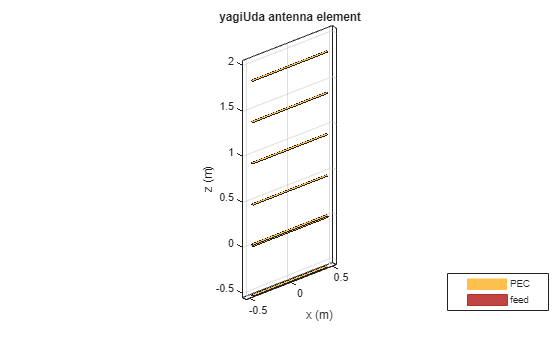### Plot Radiation Pattern at Design Frequency

Prior to executing the optimization process, plot the radiation pattern for the initial guess in 3D.

```fig1 = figure; pattern(yagidesign,fc);```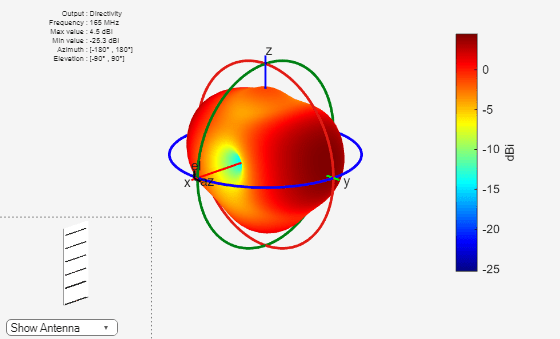This initial Yagi-Uda antenna does not have a higher directivity in the preferred direction, meaning at zenith (elevation = 90 deg) and is therefore a poorly designed radiator.

### Set Up Optimization

Use the following variables as control variables for the optimization:

• Reflector length (1 variable)

• Director lengths (4 variables)

• Reflector spacing (1 variable)

• Director spacings (4 variables)

• Exciter length (1 variable)

• Exciter spacing (1 variable)

In terms of a single vector parameter `controlVals`, set

• Reflector length = `controlVals(1)`

• Director lengths = `controlVals(2:5)`

• Reflector spacing = `controlVals(6)`

• Director spacings = `controlVals(7:10)`

• Exciter length = `controlVals(11)`

• Exciter spacing = `controlVals(12)`

In terms of `controlVals`, set an objective function that aims to have a large directivity value in the 90 degree direction, a small value in the -90 degree direction, and a large value of maximum power between the elevation beamwidth angle bounds. In addition to the directivity goal an impedance match condition is also included as a constraint. Any constraint violations will penalize the objective.

`type yagi_objective_function.m`
```function objectivevalue = yagi_objective_function(y,controlVals,fc,BW,ang,Z0,constraints) % YAGI_OBJECTIVE_FUNCTION returns the objective for a 6 element Yagi % OBJECTIVE_VALUE = % YAGI_OBJECTIVE_FUNCTION(Y,CONTROLVALS,FREQ,ANG,Z0,constraints), assigns % the appropriate parasitic dimensions, CONTROLVALS to the Yagi antenna Y, % and uses the frequency FREQ, angle pair,ANG, reference impedance Z0 and % the constraints to calculate the objective function value. % The YAGI_OBJECTIVE_FUNCTION function is used for an internal example. % Its behavior may change in subsequent releases, so it should not be % relied upon for programming purposes. % Copyright 2018 The MathWorks, Inc. y.ReflectorLength = controlVals(1); y.DirectorLength = controlVals(2:y.NumDirectors+1); y.ReflectorSpacing = controlVals(y.NumDirectors+2); y.DirectorSpacing = controlVals(y.NumDirectors+3:end-2); y.Exciter.Length = controlVals(end-1); y.Exciter.Spacing = controlVals(end); % Unpack constraints Gmin = constraints.Gmin; Gdev = constraints.Gdeviation; FBmin = constraints.FBmin; K = constraints.Penalty; % Calculate antenna port and field parameters output = analyzeAntenna(y,fc,BW,ang,Z0); % Form objective function output1 = output.MaxDirectivity+output.MismatchLoss; % Directivity/Gain at zenith % Gain constraint, e.g. G > 10 c1 = 0; if output1<Gmin c1 = Gmin-output1; end % Gain deviation constraint, abs(G-Gmin)<0.1; c1_dev = 0; if abs(output1-Gmin)>Gdev c1_dev = -Gdev + abs(output1-Gmin); end % Front to Back Ratio constraint, e.g. F/B > 15 c2 = 0; if output.FB < FBmin c2 = FBmin-output.FB; end % Form the objective + constraints objectivevalue = -output1 + max(0,(c1+c1_dev+c2))*K; end function output = analyzeAntenna(ant,fc,BW,ang,Z0) %ANALYZEANTENNA calculate the objective function % OUTPUT = ANALYZEANTENNA(Y,FREQ,BW,ANG,Z0) performs analysis on the % antenna ANT at the frequency, FC, and calculates the directivity at the % angles specified by ANG and and the front-to-back ratio. The reflection % coefficient relative to reference impedance Z0, and impedance are % computed over the bandwidth BW around FC. fmin = fc - (BW/2); fmax = fc + (BW/2); Nf = 5; freq = unique([fc,linspace(fmin,fmax,Nf)]); fcIdx = freq==fc; s = sparameters(ant,freq,Z0); Z = impedance(ant,fc); az = ang(1,:); el = ang(2,:); Dmax = pattern(ant,fc,az(1),el(1)); Dback = pattern(ant,fc,az(2),el(2)); % Calculate F/B F_by_B = Dmax-Dback; % Compute S11 and mismatch loss s11 = rfparam(s,1,1); S11 = mean((20*log10(abs(s11)))); T = max(10*log10(1 - (abs(s11(fcIdx))).^2)); % Form the output structure output.MaxDirectivity= Dmax; output.BackLobeLevel = Dback; output.FB = F_by_B; output.S11 = S11; output.MismatchLoss = T; output.Z = Z; end ```

Set bounds on the control variables.

```refLengthBounds = [0.3; % lower bound on reflector length 0.9]; % upper bound on reflector spacing dirLengthBounds = [0.3 0.3 0.3 0.3; % lower bound on director length 0.7 0.7 0.7 0.7]; % upper bound on director length refSpacingBounds = [0.05; % lower bound on reflector spacing 0.35]; % upper bound on reflector spacing dirSpacingBounds = [0.05 0.05 0.05 0.05; % lower bound on director spacing 0.2 0.25 0.3 0.3 0.2 0.2 0.3 0.3]; % upper bound on director spacing exciterLengthBounds = [0.45; % lower bound on exciter length 0.6]; % upper bound on exciter length exciterSpacingBounds = [.004; .008]; LB = [refLengthBounds(1),dirLengthBounds(1,:) refSpacingBounds(1) dirSpacingBounds(1,:) exciterLengthBounds(1) exciterSpacingBounds(1) ].*lambda; UB = [refLengthBounds(2),dirLengthBounds(2,:) refSpacingBounds(2) dirSpacingBounds(2,:) exciterLengthBounds(2) exciterSpacingBounds(2) ].*lambda; parameterBounds.LB = LB; parameterBounds.UB = UB; ang = [0 0;90 -90]; % azimuth,elevation angles for main lobe and back lobe [az;el]```

### Direct Search Based Optimization

The Global Optimization Toolbox™ provides a direct search based optimization function called `patternsearch`. We use this function with options specified with the `psoptimset` function. At every iteration, plot the best value of the objective function and limit the total number of iterations to 300. Pass the objective function to the patternsearch function by using an anonymous function together with the bounds and the options structure.The objective function used during the optimization process by `patternsearch` is available in the file `yagi_objective_function`.

The evaluation of the directivity in different directions corresponding to the angular region defined for maximum radiation as well as maximum sidelobe and the backlobe level is given in the function `calculate_objectives` available within `yagi_objective function`.

```% Optimizer options optimizerparams = optimoptions(@patternsearch); optimizerparams.UseCompletePoll = true; optimizerparams.PlotFcns = @psplotbestf; optimizerparams.UseParallel = true; optimizerparams.Cache = 'on'; optimizerparams.MaxIter = 300; optimizerparams.FunctionTolerance = 1e-2; % Antenna design parameters designparams.Antenna = yagidesign; designparams.Bounds = parameterBounds; % Analysis parameters analysisparams.CenterFrequency = fc; analysisparams.Bandwidth = BW; analysisparams.ReferenceImpedance = Z0; analysisparams.MainLobeDirection = ang(:,1); analysisparams.BackLobeDirection = ang(:,2); % Set constraints constraints.S11min = -10; constraints.Gmin = 10.5; constraints.Gdeviation = 0.1; constraints.FBmin = 15; constraints.Penalty = 50; optimdesign = optimizeAntenna(designparams,analysisparams,constraints,optimizerparams); ```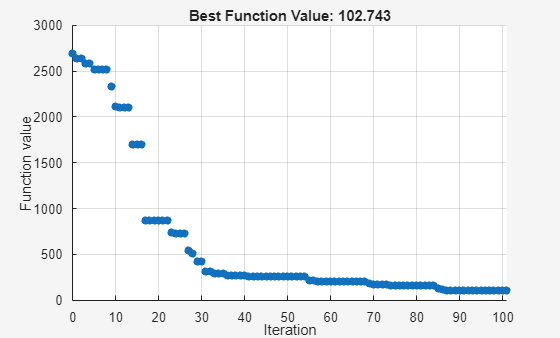```Optimization terminated: mesh size less than options.MeshTolerance. ```

### Plot Optimized Pattern

Plot the optimized antenna pattern at the design frequency.

```yagidesign.ReflectorLength = optimdesign(1); yagidesign.DirectorLength = optimdesign(2:5); yagidesign.ReflectorSpacing = optimdesign(6); yagidesign.DirectorSpacing = optimdesign(7:10); yagidesign.Exciter.Length = optimdesign(11); yagidesign.Exciter.Spacing = optimdesign(12); fig2 = figure; pattern(yagidesign,fc)```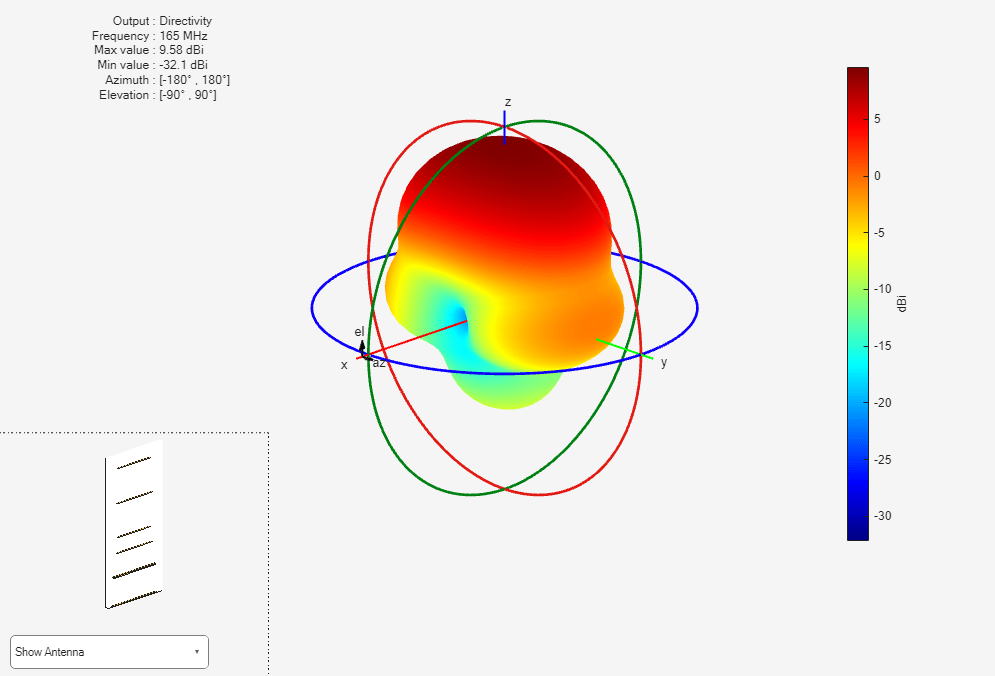### E and H-Plane Cuts of Pattern

To obtain a better insight into the behavior in the two orthogonal planes, plot the normalized magnitude of the electric field in the E and H-planes, i.e. azimuth = 0 and 90 deg respectively. Enable the antenna metrics on the polar pattern plots to establish the directivity at zenith, Front-to-Back ratio, and the beamwidth in E and H-planes.```% fig3 = figure; % patternElevation(yagidesign,fc,0,'Elevation',0:1:359); % pE = polarpattern('gco'); % pE.AntennaMetrics = 1;```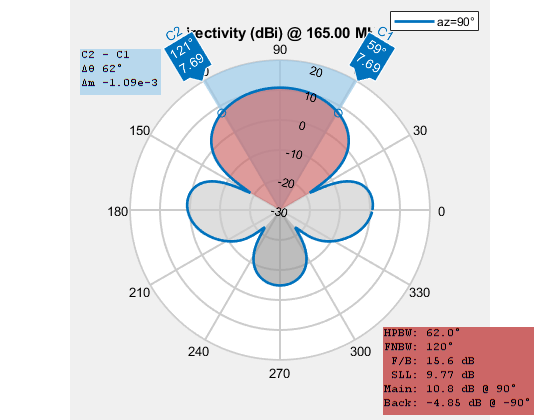```% fig4 = figure; % patternElevation(yagidesign,fc,90,'Elevation',0:1:359); % pH = polarpattern('gco'); % pH.AntennaMetrics = 1;```

The optimized design shows a significant improvement in the radiation pattern. There is higher directivity achieved in the desired direction toward zenith. The back lobe is small resulting in a good front to back ratio for this antenna.

### Input Reflection Coefficient of Optimized Antenna

The input reflection coefficient for the optimized Yagi-Uda antenna is computed and plotted relative to the reference impedance of $50\Omega$. A value of -10 dB or lower is considered to be a good impedance match.

```s = sparameters(yagidesign,freq,Z0); fig5 = figure; rfplot(s);```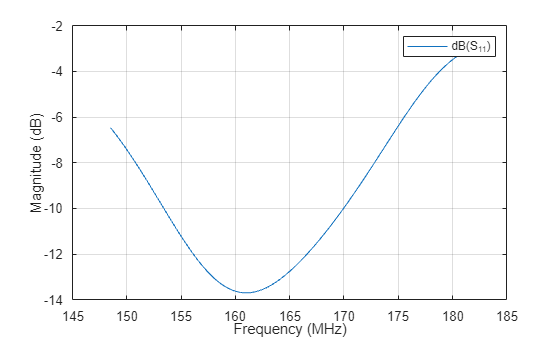### Comparison with Manufacturer Data Sheet

The optimized Yagi-Uda antenna achieves a forward directivity greater than 10 dBi, which translates to a value greater than 8 dBd (relative to a dipole). This is close to the gain value reported by the datasheet (8.5 dBd). The F/B ratio is greater than 15 dB. The optimized Yagi-Uda antenna has a E-plane and H-plane beamwidth that compare favorably to the datasheet listed values of 54 degrees and 63 degrees respectively. The design achieves a good impedance match to $300\Omega$, and has a -10 dB bandwidth of approximately 8%.

```datasheetparam = {'Gain (dBi)';'F/B';'E-plane Beamwidth (deg.)';'H-plane Beamwidth (deg.)';'Impedance Bandwidth (%)'}; datasheetvals = [10.5,16,54,63,10]'; optimdesignvals = [10.6,15.7,50,60,9]'; Tdatasheet = table(datasheetvals,optimdesignvals,'RowNames',datasheetparam)```
```Tdatasheet=5×2 table datasheetvals optimdesignvals _____________ _______________ Gain (dBi) 10.5 10.6 F/B 16 15.7 E-plane Beamwidth (deg.) 54 50 H-plane Beamwidth (deg.) 63 60 Impedance Bandwidth (%) 10 9 ```

### Tabulating Initial and Optimized Design

Tabulate the initial design guesses and the final optimized design values.

```yagiparam= {'Reflector Length'; 'Director Length - 1'; 'Director Length - 2'; 'Director Length - 3'; 'Director Length - 4'; 'Reflector Spacing'; 'Director Spacing - 1'; 'Director Spacing - 2';'Director Spacing - 3'; 'Director Spacing - 4';'Exciter Length'; 'Exciter Spacing'}; initialdesign = initialdesign'; optimdesign = optimdesign'; Tgeometry = table(initialdesign,optimdesign,'RowNames',yagiparam)```
```Tgeometry=12×2 table initialdesign optimdesign _____________ ___________ Reflector Length 0.90846 0.90846 Director Length - 1 0.90846 0.78346 Director Length - 2 0.90846 0.65846 Director Length - 3 0.90846 0.78346 Director Length - 4 0.90846 0.78346 Reflector Spacing 0.54508 0.41958 Director Spacing - 1 0.45423 0.23838 Director Spacing - 2 0.45423 0.36338 Director Spacing - 3 0.45423 0.43861 Director Spacing - 4 0.45423 0.39173 Exciter Length 0.90846 0.84602 Exciter Spacing 0.015141 0.014415 ```

### Reference

 C. A. Balanis, Antenna Theory. Analysis and Design, p. 514, Wiley, New York, 3rd Edition, 2005

 Online at: S.6Y-165